## Example Questions

← Previous 1 3

### Example Question #21 : How To Find The Solution To A Quadratic Equation

Solve for x: x2 + 4x = 5

-5 or 1

-1 or 5

-1

-5

-5 or 1

Explanation:

Solve by factoring.  First get everything into the form Ax2 + Bx + C = 0:

x2 + 4x - 5 = 0

Then factor: (x + 5) (x - 1) = 0

Solve each multiple separately for 0:

X + 5 = 0; x = -5

x - 1 = 0; x = 1

Therefore, x is either -5 or 1

### Example Question #1 : Quadratic Equation

Solve for x: (x2 – x) / (x – 1) = 1

x = -1

x = 2

x = 1

x = -2

No solution

No solution

Explanation:

Begin by multiplying both sides by (x – 1):

x2 – x = x – 1

Solve as a quadratic equation: x2 – 2x + 1 = 0

Factor the left: (x – 1)(x – 1) = 0

Therefore,  x = 1.

However, notice that in the original equation, a value of 1 for x would place a 0 in the denominator.  Therefore, there is no solution.

### Example Question #1 : Quadratic Equation

x2 – 3x – 18 = 0

Quantity A: x

Quantity B: 6

B. Quantity B is greater.

A. Quantity A is greater

D. The relationship cannot be determined from the information given.

C. The two quantities are equal.

D. The relationship cannot be determined from the information given.

Explanation:

x2 – 3x  18 = 0 can be factored to ( 6)(x + 3) = 0

Therefore, x can be either 6 or 3.

### Example Question #1 : Quadratic Equation

A farmer has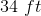of fence, and wants to fence in his sheep. He wants to build a rectangular pen with one side formed by the side of his barn. He wants the area of the pen to be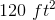. Which of the following is a possible dimension for the side opposite the barn?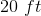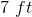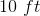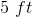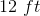Explanation:

Set up two equations from the given information: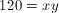and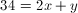.

Substitute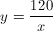into the second equation: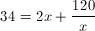Multiply through by.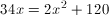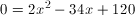Divide by 2: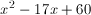We can factor this: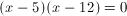Thus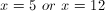.

Note that this is not the side opposite the barn. We need to solve for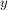in both cases, getting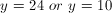.

24 does not appear in the choices, so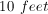is the only possible correct answer.

### Example Question #1 : Quadratic Equations

What is the sum of all the values ofthat satisfy: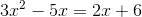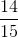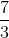Explanation:

With quadratic equations, always begin by getting it into standard form:Therefore, take our equation:And rewrite it as: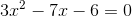You could use the quadratic formula to solve this problem.  However, it is possible to factor this if you are careful.  Factored, the equation can be rewritten as: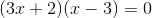Now, either one of the groups on the left could beand the whole equation would be.  Therefore, you set up each as a separate equation and solve for: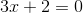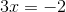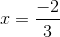OR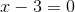The sum of these values is: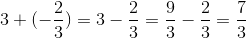### Example Question #1 : How To Find The Solution To A Quadratic Equation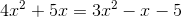Quantity A:Quantity B:Quantity A is larger.

A comparison cannot be detemined from the given information.

The two quantities are equal.

Quantity B is larger.

Quantity B is larger.

Explanation:

With quadratic equations, always begin by getting it into standard form: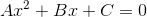Therefore, take our equation:And rewrite it as: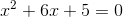Now, while you could use the quadratic formula to solve this problem, the easiest way to work this question is to factor the left side of the equation.  This gives you: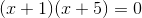Now, either one of the groups on the left could beand the whole equation would be.  Therefore, you set up each as a separate equation and solve for: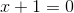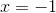OR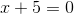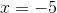Since both of your answers are less than, quantity B is larger than quantity A.

### Example Question #1 : How To Find The Solution To A Quadratic Equation

If f(x) = -x2 + 6x - 5, then which could be the value of a if f(a) = f(1.5)?

2.5
4
3.5
4.5
1
Explanation:

We need to input 1.5 into our function, then we need to input "a" into our function and set these results equal.

f(a) = f(1.5)

f(a) = -(1.5)2 +6(1.5) -5

f(a) = -2.25 + 9 - 5

f(a) = 1.75

-a2 + 6a -5 = 1.75

Multiply both sides by 4, so that we can work with only whole numbers coefficients.

-4a2 + 24a - 20 = 7

Subtract 7 from both sides.

-4a2 + 24a - 27 = 0

Multiply both sides by negative one, just to make more positive coefficients, which are usually easier to work with.

4a2 - 24a + 27 = 0

In order to factor this, we need to mutiply the outer coefficients, which gives us 4(27) = 108. We need to think of two numbers that multiply to give us 108, but add to give us -24. These two numbers are -6 and -18. Now we rewrite the equation as:

4a2 - 6a -18a + 27 = 0

We can now group the first two terms and the last two terms, and then we can factor.

(4a2 - 6a )+(-18a + 27) = 0

2a(2a-3) + -9(2a - 3) = 0

(2a-9)(2a-3) = 0

This means that 2a - 9 =0, or 2a - 3 = 0.

2a - 9 = 0

2a = 9

a = 9/2 = 4.5

2a - 3 = 0

a = 3/2 = 1.5

So a can be either 1.5 or 4.5.

The only answer choice available that could be a is 4.5.

### Example Question #1 : Quadratic Equations

Solve for x:  2(x + 1)2 – 5 = 27

–2 or 5

–3 or 2

3 or –5

3 or 4

–2 or 4

3 or –5

Explanation:

Quadratic equations generally have two answers.  We add 5 to both sides and then divide by 2 to get the quadratic expression on one side of the equation: (x + 1)2 = 16.   By taking the square root of both sides we get x + 1 = –4 or x + 1 = 4.  Then we subtract 1 from both sides to get x = –5 or x = 3.

### Example Question #2 : Quadratic Equation

Two consecutive positive multiples of three have a product of 54. What is the sum of the two numbers?

6

15

3

9

12

15

Explanation:

Define the variables to be x = first multiple of three and x + 3 = the next consecutive multiple of 3.

Knowing the product of these two numbers is 54 we get the equation x(x + 3) = 54. To solve this quadratic equation we need to multiply it out and set it to zero then factor it. So x2 + 3x – 54 = 0 becomes (x + 9)(x – 6) = 0.  Solving for x we get x = –9 or x = 6 and only the positive number is correct.  So the two numbers are 6 and 9 and their sum is 15.

### Example Question #11 : Quadratic Equations

Solve 3x2 + 10x = –3

x = –1/9 or –9

x = –2/3 or –2

x = –4/3 or –1

x = –1/3 or –3

x = –1/6 or –6

x = –1/3 or –3

Explanation: